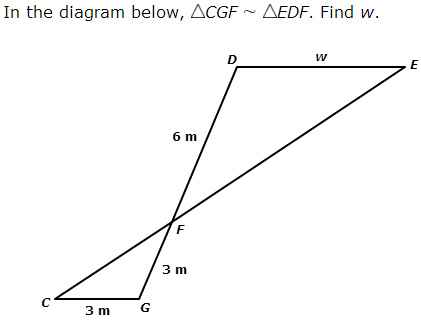Similar Figures
3 years ago
mrswatt206
Save
Edit
Host a game
Live GameLive
Homework
Solo Practice
Practice14 QuestionsShow answers
• Question 1
180 seconds
Q. Matching sides are called
Corresponding Sides
Corresponding Angles
The Same
Congruent Sides
• Question 2
60 seconds
Q. All angles in similar figures are congruent.
True
False
• Question 3
180 secondsQ. Are the following similar? Why or why not?
Yes
No, the corresponding angles are not equal.
No, the ratios of the corresponding sides are not equal.
• Question 4
180 secondsQ. The pair of figures is similar. Find the missing side.
x = 20
x = 12
x = 5
x = 4
• Question 5
180 secondsQ. To find the height of a very tall pine tree, you place a mirror on the ground and stand where you can see the top of the pine tree.  How tall is the tree?
144 feet
72 feet
36 feet
• Question 6
300 seconds
Q. Gwen scans a photo that is 4 in. wide by 6 in. tall into her computer. If she scales the height down to 3 in., how wide should the similar photo be?
3 in
2 in.
4 in
1 in
• Question 7
300 seconds
Q. A rectangular garden is 45 ft wide and 70 ft long. On a blueprint, the width is 9 in. Identify the length on the blueprint.
7 in
9 in
12 in
14 in
• Question 8
30 secondsQ. Find the perimeter of the large triangle.
24
36
12
54
• Question 9
180 secondsQ. Complete each proportion.
CB / DM  =  AC / ?
BM
MA
BC
• Question 10
60 secondsQ. The two right triangles below are similar. What is x, the missing side length in triangle DEF?
3.75
9.75
10
12
• Question 11
180 secondsQ. Review the picture.  Find the missing side measurement in the picture using a proportion.
w = 18 m
w = 6 m
6 m
w = 0.16 m
• Question 12
180 secondsQ. Find the missing perimeter.
15
20
25
30
• Question 13
180 secondsQ. Find the missing area.
0
12
24
30
• Question 14
180 secondsQ. Find the missing area.